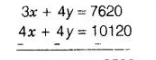# A railway half ticket cost half the full fare but the reservation

Question:

A railway half ticket cost half the full fare but the reservation charges are the same on a half ticket as on a full ticket. One reserved first class ticket from

the stations A to B costs ₹ 2530. Also, one reserved first class ticket and one reserved first class half ticket from stations A to B costs ₹ 3810. Find the

full first class fare from stations A to B and also the reservation charges for a ticket.

Solution:

Let the cost of full and half first class fare be $₹ \frac{X}{2}$ and $₹$ respectively and reservation charges be $₹$ y per ticket.

Case I The cost of one reserved first class ticket from the stations $A$ to $B$

$=₹ 2530$

$\Rightarrow \quad x+y=2530$ ....(i)

Case II The cost of one reserved first class ticket and one reserved first class half ticket from stations $A$ to $B=₹ 3810$

$\Rightarrow \quad x+y+\frac{x}{2}+y=3810$

$\Rightarrow \quad \frac{3 x^{2}}{2}+2 y=3810$

$\Rightarrow \quad 3 x+4 y=7620$ ...(ii)

Now, multiplying Eq. (i) by 4 and then subtracting from Eq. (ii), we get$-x=-2500$

$\Rightarrow \quad x=2500$

On putting the value of $x$ in Eq. (i), we get

$2500+v=2530$

$\Rightarrow \quad y=2530-2500$

$\Rightarrow \quad y=30$

Hence, full first class fare from stations A to 6 is ₹ 2500 and the reservation for a ticket is ₹30.